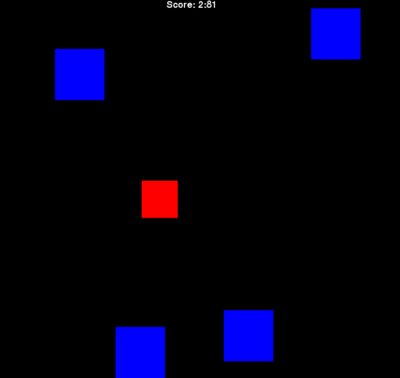# Escape

dodge the boxes as long as you can.Jamie
(jboisture)
My first Pygame game. Just try to dodge the blue boxes as long as you can.

### Changes### Releases

•#### Justin 2012-12-10 16:57

I am trying to make a similar program to this one and came across your game that has about the same Idea as mine. I just can't get the collision detection to work. If you are still on this site do you think you can help a little bit?

import pygame, sys, random, time
from pygame.locals import *

# set up pygame
pygame.init()
mainClock = pygame.time.Clock()

# set up the window
WINDOWWIDTH = 1000
WINDOWHEIGHT = 1000
windowSurface = pygame.display.set_mode((WINDOWWIDTH, WINDOWHEIGHT), 0, 32)
pygame.display.set_caption('Final')

BLACK = (0, 0, 0)
WHITE = (255, 255, 255)
RED = (255, 0, 0)
NEWBLOCK = 40
BLOCKSIZE = 20
player = pygame.Rect(500, 500, 30, 30)
blocks = []

moveLeft = False
moveRight = False
moveUp = False
moveDown = False

MOVESPEED = 7

DOWNLEFT = 1
DOWNRIGHT = 3
UPLEFT = 7
UPRIGHT = 9

b1 ={'rect':pygame.Rect(1, 1, 50, 50), 'color':RED, 'dir':UPLEFT}
b2 ={'rect':pygame.Rect(925, 1, 50, 50), 'color':RED, 'dir':UPRIGHT}
b3 ={'rect':pygame.Rect(760, 760, 50, 50), 'color':RED, 'dir':UPLEFT}
b4 ={'rect':pygame.Rect(1, 925, 50, 50), 'color':RED, 'dir':UPRIGHT}
b5 ={'rect':pygame.Rect(1, 462, 50, 50), 'color':RED, 'dir':UPLEFT}
b6 ={'rect':pygame.Rect(462, 231, 50, 50), 'color':RED, 'dir':UPRIGHT}
b7 ={'rect':pygame.Rect(231, 462, 50, 50), 'color':RED, 'dir':UPLEFT}
b8 ={'rect':pygame.Rect(325, 325, 50, 50), 'color':RED, 'dir':UPLEFT}
b9 ={'rect':pygame.Rect(100, 625, 50, 50), 'color':RED, 'dir':UPLEFT}
b10 ={'rect':pygame.Rect(530, 1, 50, 50), 'color':RED, 'dir':UPLEFT}
blocks = [b1, b2, b3, b4, b5, b6, b7, b8, b9, b10]

while True:
# check for events
for event in pygame.event.get():
if event.type == QUIT:
pygame.quit()
sys.exit()
if event.type == KEYDOWN:
# change the keyboard variables
if event.key == K_LEFT or event.key == ord('a'):
moveRight = False
moveLeft = True
if event.key == K_RIGHT or event.key == ord('d'):
moveLeft = False
moveRight = True
if event.key == K_UP or event.key == ord('w'):
moveDown = False
moveUp = True
if event.key == K_DOWN or event.key == ord('s'):
moveUp = False
moveDown = True
if event.type == KEYUP:
if event.key == K_ESCAPE:
pygame.quit()
sys.exit()
if event.key == K_LEFT or event.key == ord('a'):
moveLeft = False
if event.key == K_RIGHT or event.key == ord('d'):
moveRight = False
if event.key == K_UP or event.key == ord('w'):
moveUp = False
if event.key == K_DOWN or event.key == ord('s'):
moveDown = False

for b in blocks:
# move the block data structure
if b['dir'] == DOWNLEFT:
b['rect'].left -= MOVESPEED
b['rect'].top += MOVESPEED
if b['dir'] == DOWNRIGHT:
b['rect'].left += MOVESPEED
b['rect'].top += MOVESPEED
if b['dir'] == UPLEFT:
b['rect'].left -= MOVESPEED
b['rect'].top -= MOVESPEED
if b['dir'] == UPRIGHT:
b['rect'].left += MOVESPEED
b['rect'].top -= MOVESPEED
# check if the block has move out of the window
if b['rect'].top < 0:
# block has moved past the top
if b['dir'] == UPLEFT:
b['dir'] = DOWNLEFT
if b['dir'] == UPRIGHT:
b['dir'] = DOWNRIGHT
if b['rect'].bottom > WINDOWHEIGHT:
# block has moved past the bottom
if b['dir'] == DOWNLEFT:
b['dir'] = UPLEFT
if b['dir'] == DOWNRIGHT:
b['dir'] = UPRIGHT
if b['rect'].left < 0:
# block has moved past the left side
if b['dir'] == DOWNLEFT:
b['dir'] = DOWNRIGHT
if b['dir'] == UPLEFT:
b['dir'] = UPRIGHT
if b['rect'].right > WINDOWWIDTH:
# block has moved past the right side
if b['dir'] == DOWNRIGHT:
b['dir'] = DOWNLEFT
if b['dir'] == UPRIGHT:
b['dir'] = UPLEFT
# draw the block onto the surface
pygame.draw.rect(windowSurface, b['color'], b['rect'])

pygame.display.update()
time.sleep(0.02)

windowSurface.fill(BLACK)
if moveDown and player.bottom < WINDOWHEIGHT:
player.top += MOVESPEED
if moveUp and player.top > 0:
player.top -= MOVESPEED
if moveLeft and player.left > 0:
player.left -= MOVESPEED
if moveRight and player.right < WINDOWWIDTH:
player.right += MOVESPEED

for b in blocks[:]:
if player.colliderect(players):
players.remove(player)

pygame.draw.rect(windowSurface, WHITE, player)